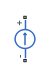# Current Source

Current source with optional DC, AC, and noise components

• Library:
• Simscape / Electrical / Sources

•## Description

The Current Source block implements a current source with DC, AC, and noise components. The current flowing through the source from the terminal to the + terminal is given by:

`$i={i}_{DC}+{i}_{AC}\mathrm{sin}\left(2\pi ft+\varphi \right)+{i}_{N}$`

where:

• iDC is the steady-state DC current component.

• iAC is the amplitude of the AC current component.

• f is the frequency of the AC component.

• ϕ is the phase offset of the AC component.

• iN is the noise current.

You can configure your source as DC-only, AC-only, or a combination of both. By default, both AC and DC components are set to 0. Define the AC/DC current by specifying nonzero parameter values after placing the block in your model.

The noise component is also optional. If you set the Noise mode parameter to `Enabled`, then the added noise current is given by:

`${i}_{N}=\sqrt{{P}_{i}/2}\frac{N\left(0,1\right)}{\sqrt{h}}$`

where:

• Pi is the single-sided noise power spectral density for a 1 ohm load, in A^2/Hz.

• N is a Gaussian random number with zero mean and standard deviation of one.

• h is the sampling interval.

By default, the Noise mode parameter is set to `Disabled`, and the current source generates no thermal noise.

### Noise Options

The block generates Gaussian noise by using the PS Random Number source in the Simscape™ Foundation library. You can control the random number seed by setting the Repeatability parameter:

• `Not repeatable` — Every time you simulate your model, the block resets the random seed using the MATLAB® random number generator:

`seed = randi(2^32-1);`
• `Repeatable` — The block automatically generates a seed value and stores it inside the block, to always start the simulation with the same random number. This auto-generated seed value is set when you add a Current Source block from the block library to the model. When you make a new copy of the Current Source block from an existing one in a model, a new seed value is generated. The block sets the value using the MATLAB random number generator command shown above.

• `Specify seed` — If you select this option, the additional Seed parameter lets you directly specify the random number seed value.

## Assumptions and Limitations

• Simulating with noise enabled slows down simulation. Choose the sample time (h) so that noise is generated only at frequencies of interest, and not higher.

## Ports

### Conserving

expand all

Electrical conserving port associated with the current source positive terminal

Electrical conserving port associated with the current source negative terminal

## Parameters

expand all

### DC & AC Components

DC component of the output current. Specify a nonzero value to add a DC component to the current source.

Amplitude of the AC component of the output current. Specify a nonzero value to add an AC component to the current source.

Phase offset of the AC component of the output current.

Frequency of the AC component of the output current.

### Noise

Select the noise option:

• `Disabled` — No noise is produced by the current source. This is the default.

• `Enabled` — The current source generates thermal noise, and the associated parameters become visible on the Noise tab.

The single-sided spectrum noise power. Strictly-speaking, this is a density function for the square of the current, commonly thought of as a power into a 1 ohm load, and therefore units are A^2/Hz. To avoid this unit ambiguity, some datasheets quote noise current as a noise density with units of A/√Hz. In this case, you should enter the square of the noise density quoted in the datasheet as the parameter value.

Select the noise control option:

• `Not repeatable` — The random sequence used for noise generation is not repeatable. This is the default.

• `Repeatable` — The random sequence used for noise generation is repeatable, with a system-generated seed.

• `Specify seed` — The random sequence used for noise generation is repeatable, and you control the seed by using the Seed parameter.

Random number seed stored inside the block to make the random sequence repeatable. The parameter value is automatically generated using the MATLAB random number generator command. You can modify this parameter value, but it gets overwritten by a new random value if you copy the block to another block in the model. Therefore, if you want to control the seed of the random sequence, use the `Specify seed` option for the Repeatability parameter and specify the desired seed value using the Seed parameter.

#### Dependencies

This parameter is visible only when you select `Repeatable` for the Repeatability parameter.

Random number seed used by the noise random number generator.

#### Dependencies

This parameter is visible only when you select ```Specify seed``` for the Repeatability parameter.

Defines the rate at which the noise source is sampled. Choose it to reflect the frequencies of interest in your model. Making the sample time too small will unnecessarily slow down your simulation.

## Version History

Introduced in R2013a